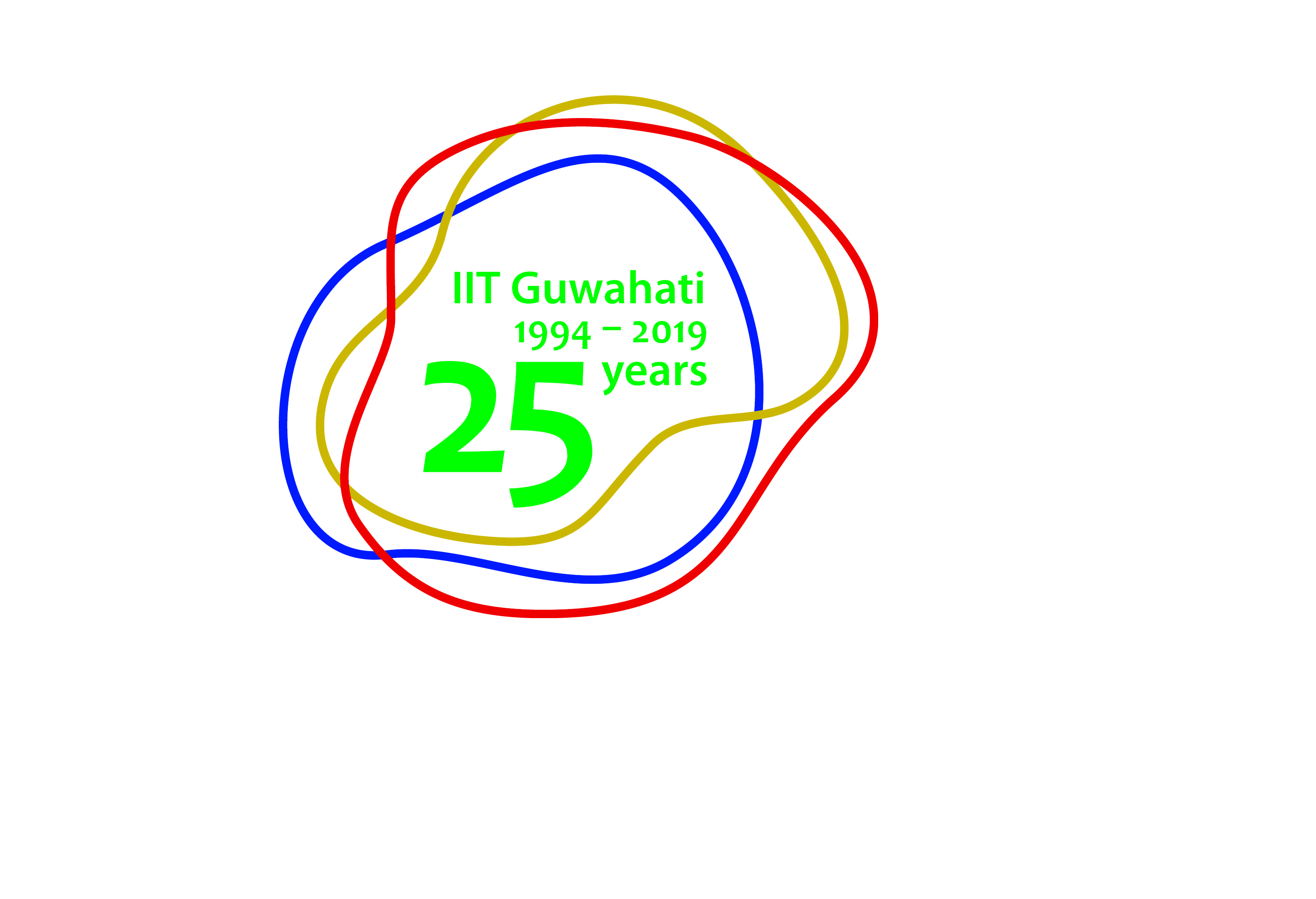﻿ Energy | Services# School of Energy Science and Engineering

#### Indian Institute of Technology Guwahati

Revised sample testing rates (WEF 17/06/21):

For analysis of external sample, registration forms (Job order form) are available at the Office of the School of Energy Science and Engineering or can be downloaded from the link given below. The filled in registration form along with the requisite testing charge in the form of A/C payee cheque or DD favouring "IIT Guwahati" must be deposited to the Head, School of Energy Science and Engineering. The sample analysis charges are listed below -

 Type of Analysis Sample charge (In INR) Academic Institutes Govt R&D Organizations Private firms/Industries Calorific value analysis by Bomb Calorimeter Rs. 1000/- per sample Rs. 1250/- per sample Rs. 1500/- per sample Thermo Gravimatric analysis (TGA) Rs. 1500/- per sample Rs. 2000/- per sample Rs. 2500/- per sample Gas Chromatograph (GC) analysis Rs. 1500/- per sample Rs. 2000/- per sample Rs. 2500/- per sample Viscosity measurement Rs. 1000/- per sample Rs. 1250/- per sample Rs. 1500/- per sample Flash and fire point measurement Rs. 1000/- per sample Rs. 1250/- per sample Rs. 1500/- per sample Cloud and pour point measurement Rs. 1500/- per sample Rs. 2000/- per sample Rs. 2500/- per sample Proximate Analysis Rs. 2000/- per sample Rs. 3000/- per sample Rs. 4000/- per sample Exhaust Gas Analysis Rs. 3000/- per sample Rs. 4000/- per sample Rs. 5000/- per sample HPLC Analysis Rs. 2000/- per sample Rs. 3000/- per sample Rs. 4000/- per sample